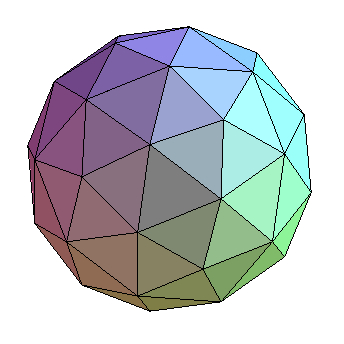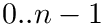Structural Bioinformatics Library Template C++ / Python API for developping structural bioinformatics applications.
User Manual# Triangulations_extensions_3

Authors: F. Cazals and T. Dreyfus

# Introduction

This package provides constructions, predicates and Output / Input functionality related to the three dimensional triangulations data structures of CGAL. Three kind of triangulations are concerned:

• Triangulation_3, that is a simple 3D triangulation of a set of points,
• Delaunay_triangulation_3, that is a 3D Delaunay triangulation of a set of points,
• Regular_triangulation_3, that is a 3D triangulation of a set of multiplicative weighted points.

In the following, we describe more precisely the provided functionality for each kind of triangulation.

## Triangulation_3

Four main functionality are provided:

• The canonical representation of the edges and facets. The edges of the CGAL Triangulation_3 are represented by a cell bounded by this edge and the indices of the two vertices in the cell bounding the edge. The facets of the CGAL Triangulation_3 are represented by a cell bounded by this facet and the index of the vertex in the cell that does not bound the facet. Both representations are not unique since an edge or a facet may bound several cells. For the edges, we can canonically represent an edge with the two bounding vertices ordered with a lexicographical order (assuming that there is an order over the vertices). For the facets, we can canonically represent a facet with the cell that compares the lesser (assuming that there is an order over the cells). These representations allow in particular to manipulate container of canonical edges and facets requiring a total order over its elements.
• The manipulation of faces and cofaces of a simplex: we provide three functors allowing to (i) access to the two edges of a given facet that are not equal to a given edge, (ii) access to the facet common to two given cells, and (iii) check that a given simplex is the co-face of another given simplex.
• The serialization of the whole triangulation: it consists to save into an archive (a xml file for example) the simplices of the triangulation, and to load a triangulation from an archive.
• The print of the triangulation onto a VMD file format: it consists to save the triangulation into a file readable by the VMD software.

See the Triangulation_extension_3 Example section for an example.

## Delaunay_triangulation_extension_3

This extension provides functors for accessing statistics on the dual of the edges and facets of a Delaunay triangulation:

• for the edges, one functor constructs its dual Voronoi face as a sequence of points, while the other functor constructs the area (possibly infinite) of the dual Voronoi face.
• for the facets, there is only one functor that constructs the squared length (possibly infinite) of a Voronoi dual edge, the original Delaunay triangulation of CGAL providing the construction of the dual Voronoi edge.

See the Delaunay_triangulation_extension_3 Example section for an example.

## Regular_triangulation_extension_3

See the Regular_triangulation_extension Example section for an example.

# Implementation

Each extension is represented by one class designed as a traits class defining the functors corresponding to the different constructions and predicates:

• SBL::GT::T_Regular_triangulation_extension_3< RegularTriangulation3 >, where the template parameter corresponds to the RegularTriangulation3 concept of CGAL.

Furthermore, there data structures allowing to serialize in an archive, or to print in a VMD file format, the simplices of:

• a 3D Triangulation: SBL::GT::T_Triangulation_3_serialization_output_traits< Triangulation3 > and SBL::GT::T_Triangulation_3_VMD_output_traits< Triangulation3 >,
• a 3D Delaunay Triangulation: SBL::GT::T_Delaunay_triangulation_3_serialization_output_traits< DelaunayTriangulation3 > and SBL::GT::T_Delaunay_triangulation_3_VMD_output_traits< DelaunayTriangulation3 >,
• a 3D Regular Triangulation: SBL::GT::T_Regular_triangulation_3_serialization_output_traits< RegularTriangulation3 > and SBL::GT::T_Regular_triangulation_3_VMD_output_traits< RegularTriangulation3 >.

## Dependencies

This package has one internal dependency, that is the Spherical_kernel_extension_3 package for the use of the SBL::GT::T_Spherical_kernel_extension_3::Is_counter_clockwise_oriented predicate .

There are also two external dependencies:

• the CGAL library, for the representation of 3D Triangulations and-complexes. Note that this part of the CGAL library is generic and consists only of C++ header files.
• the Boost.Serialization library, for serializing the simplices of a 3D Triangulation. Note that this part of the Boost library is not generic and requires to be linked during the compilation of a program using the serialized data structures. However, if you are not using the serialized data structures, you can skip this step, even if you are using this package.

# Examples

## Triangulation_extension_3 Example

The following example presents the class SBL::GT::T_Triangulation_extension_3 with a simple triangulation from CGAL. It reads a file containing three dimensional points and insert them in the triangulation. Then, it stores and sorts all the facets of the triangulation. Then two predicates from the SBL::GT::T_Triangulation_extension_3 class are applied to the smallest facet.

#include <iostream>
#include <fstream>
#include <set>
#include <SBL/GT/Triangulation_extension_3.hpp>
#include <CGAL/Exact_predicates_inexact_constructions_kernel.h>
#include <CGAL/Triangulation_3.h>
typedef CGAL::Exact_predicates_inexact_constructions_kernel K;
typedef CGAL::Triangulation_3<K> Triangulation_3;
typedef Triangulation_3::Cell_handle Cell_handle;
typedef Triangulation_3::Facet Facet;
//Extension for the 3D Triangulation.
//Canonical representation of facets of the triangulation.
typedef Extension::Canonical_facet Canonical_facet;
int main(int argc, char *argv[])
{
Triangulation_3 T;
//Read 3D point from an input file and insert them in the
//triangulation.
if(argc < 2) return -1;
std::ifstream in(argv);
while(!in.eof())
{
K::Point_3 p;
in >> p;
if(in.eof()) continue;
unsigned n; in >> n;
T.insert(p);
}
in.close();
//Collect the facets and sort them using their canonical representation.
std::set<Canonical_facet> facets;
for(Triangulation_3::Finite_facets_iterator it = T.finite_facets_begin();
it != T.finite_facets_end(); it++)
facets.insert(Canonical_facet(*it, T));
std::cout << "Number of finite facets in the triangulation: " << facets.size() << std::endl;
//Check that the cells bounding a facet (here the first one of the
//collected facets) are cofaces of this facet.
Extension::Is_coface is_coface;
Cell_handle c_1 = facets.begin()->get_facet().first;
Cell_handle c_2 = facets.begin()->get_facet().first->neighbor(facets.begin()->get_facet().second);
std::cout << "Incident cells are cofaces of a facet: "
<< (is_coface(c_1, facets.begin()->get_facet()) &&
is_coface(c_2, facets.begin()->get_facet())) << std::endl;
//Check that the common facet f' of the bounding cells of a facet f is f.
Extension::Get_common_facet get_common_facet;
Facet f = get_common_facet(c_1, c_2);
std::cout << "Common facet of incident cells is the facet itself: "
<< (Canonical_facet(f, T) == *facets.begin()) << std::endl;
return 0;
}
Defines simple predicates and constructions structure over a triangulation.
Definition: Triangulation_extension_3.hpp:646
Extension completing the 3D Spherical kernel of CGAL.
Definition: Spherical_kernel_extension_3.hpp:104
p
Definition: extract-wales-graph.py:74
dictionary T
Definition: extract-wales-graph.py:35
n
Definition: extract-wales-graph.py:70
f
Definition: generate-random-balls-3.py:21

## Delaunay_triangulation_extension_3 Example

The following example presents the class SBL::GT::T_Delaunay_triangulation_extension_3 with a Delaunay triangulation from CGAL. It reads a file containing three dimensional points and insert them in the triangulation. Then, it compute the area of the dual of all finite edges in the triangulation.

#include <iostream>
#include <fstream>
#include <SBL/GT/Delaunay_triangulation_extension_3.hpp>
#include <CGAL/Exact_predicates_inexact_constructions_kernel.h>
#include <CGAL/Delaunay_triangulation_3.h>
//Instantiation of a 3D Delaunay triangulation with CGAL, using
//rational number types.
typedef CGAL::Exact_predicates_inexact_constructions_kernel K;
typedef CGAL::Delaunay_triangulation_3<K> Triangulation_3;
//Extension for the 3D Delaunay triangulation.
int main(int argc, char *argv[])
{
Triangulation_3 T;
//Read 3D point from an input file and insert them in the
//triangulation.
if(argc < 2) return -1;
std::ifstream in(argv);
while(!in.eof())
{
K::Point_3 p;
in >> p;
if(in.eof()) continue;
unsigned n; in >> n;
T.insert(p);
}
in.close();
//Compute the area of the dual of all finite edges of the triangulation.
Extension::Get_area_of_dual_of_edge get_area(T);
for(Triangulation_3::Finite_edges_iterator it = T.finite_edges_begin();
it != T.finite_edges_end(); it++)
std::cout << "area of dual of edge: " << get_area(*it)<< std::endl;
return 0;
}
Defines simple predicates and constructions structure over a triangulation.
Definition: Delaunay_triangulation_extension_3.hpp:112

## Regular_triangulation_extension Example

The following example presents the class SBL::GT::T_Regular_triangulation_extension_3 with a 3D regular triangulation from CGAL. It reads a file containing three dimensional spheres and insert them in a weighted-complex. Then, for each finite cell with a regular or singular facet, it checks the orientation of this facet.

#include <iostream>
#include <fstream>
#include <CGAL/Exact_predicates_inexact_constructions_kernel.h>
#include <CGAL/Regular_triangulation_euclidean_traits_3.h>
#include <CGAL/Regular_triangulation_3.h>
#include <SBL/IO/VMD_viewer.hpp>
#include <SBL/IO/Regular_triangulation_3_molecular_view.hpp>
typedef CGAL::Exact_predicates_inexact_constructions_kernel K;
typedef CGAL::Regular_triangulation_euclidean_traits_3<K> RTraits;
typedef CGAL::Triangulation_vertex_base_3<RTraits> Vb;
typedef CGAL::Regular_triangulation_cell_base_3<RTraits> Cb;
typedef CGAL::Triangulation_data_structure_3<Vb, Cb> Tds;
typedef CGAL::Regular_triangulation_3<RTraits, Tds> Regular_triangulation_3;
int main(int argc, char *argv[])
{
Regular_triangulation_3 T;
//Read 3D point from an input file and insert them in the
//triangulation.
if(argc < 2) return -1;
std::ifstream in(argv);
while(!in.eof())
{
CGAL::Weighted_point<K::Point_3, K::FT> wp;
in >> wp;
if(in.eof()) continue;
T.insert(wp);
}
in.close();
std::ofstream out("triangulation.vmd");
SBL::IO::VMD_viewer viewer(out);
viewer << std::make_pair("Triangulation", &T);
out.close();
return 0;
}
Viewer writing in VMD file format.
Definition: VMD_viewer.hpp:346